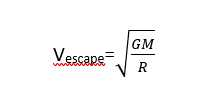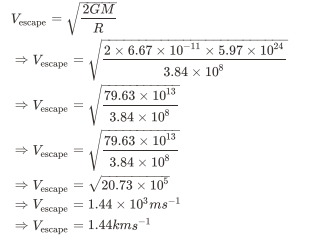# ﻿Why does the moon revolve around the earth but not the sun? (even if the gravitational force between the sun and moon is twice of the gravitational force between the earth and the moon.)

﻿Why does the moon revolve around the earth but not the sun? (even if the gravitational force between the sun and moon is twice of the gravitational force between the earth and the moon.)

428

We know that the gravitational force of attraction keeps the moon revolving around the earth, and the earth revolving around the Sun. However, recall that for the moon to escape the bound of the earth’s gravitational force and fall under the influence of the Sun’s gravity, it must possess a certain velocity. This is what is known as escape velocity.

Determine a relation for the escape velocity by equating the centripetal force of attraction between the moon and the earth and the gravitational force acting between them and arrive at a numerical value for the escape velocity. Keeping in mind that the revolutionary speed of the moon is 1.02 km.s-1

The revolution of the moon around the earth is due to gravitational force. The sun also exerts gravitational force on the moon, which is almost twice as large as the gravitational force of earth. But even after this the moon revolves around the earth. To understand this, we need to know the concept of escape velocity. The escape velocity of an object can be defined as the velocity required for an object to overcome the gravitational attraction of the planet or any star.

The mathematical expression for escape velocity can be given as,Where G is the gravitational constant, M is the mass of earth and R is the distance of the object from the centre of earth.

Now, G = 6.67 × 10 k-11m3 g -1s-2

M = 5.97 × 1024 kg

Distance of moon from earth,

R = 3.84 × 105km

So, escape velocity of moon from earth’s surface is,So, the velocity of moon to escape earth and revolve around the sun is approximately,

Vescape=1.44kms-1

But the velocity of moon around earth is V = 1.02kms-1

Which is lower than the escape velocity needed for the moon to go out of earth’s gravitational pull.

Because of this the moon revolves around earth, not sun.